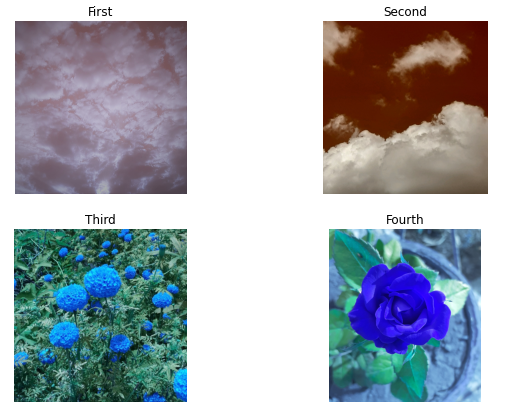Related Articles

# How to Display Multiple Images in One Figure Correctly in Matplotlib?

• Last Updated : 02 Feb, 2021

The easiest way to display multiple images in one figure is use figure(), add_subplot(), and imshow() methods of Matplotlib. The approach which is used to follow is first initiating fig object by calling fig=plt.figure() and then add an axes object to the fig by calling add_subplot() method. Then will display the image using imshow() method.

Here rows and columns are the total number of rows and columns in the figure and i is the position at which new subplot must be placed.

Steps:

• Import required libraries
• Create a figure
• Set values of rows and column variables
• Add subplot and display image one by one

Consider below Images used as input:Image1Image2Image3Image4

Below is the implementation :

## Python3

 `# code for displaying multiple images in one figure`` ` `#import libraries``import` `cv2``from` `matplotlib ``import` `pyplot as plt`` ` `# create figure``fig ``=` `plt.figure(figsize``=``(``10``, ``7``))`` ` `# setting values to rows and column variables``rows ``=` `2``columns ``=` `2`` ` `# reading images``Image1 ``=` `cv2.imread(``'Image1.jpg'``)``Image2 ``=` `cv2.imread(``'Image2.jpg'``)``Image3 ``=` `cv2.imread(``'Image3.jpg'``)``Image4 ``=` `cv2.imread(``'Image4.jpg'``)`` ` `# Adds a subplot at the 1st position``fig.add_subplot(rows, columns, ``1``)`` ` `# showing image``plt.imshow(Image1)``plt.axis(``'off'``)``plt.title(``"First"``)`` ` `# Adds a subplot at the 2nd position``fig.add_subplot(rows, columns, ``2``)`` ` `# showing image``plt.imshow(Image2)``plt.axis(``'off'``)``plt.title(``"Second"``)`` ` `# Adds a subplot at the 3rd position``fig.add_subplot(rows, columns, ``3``)`` ` `# showing image``plt.imshow(Image3)``plt.axis(``'off'``)``plt.title(``"Third"``)`` ` `# Adds a subplot at the 4th position``fig.add_subplot(rows, columns, ``4``)`` ` `# showing image``plt.imshow(Image4)``plt.axis(``'off'``)``plt.title(``"Fourth"``)`

Output:Attention geek! Strengthen your foundations with the Python Programming Foundation Course and learn the basics.

To begin with, your interview preparations Enhance your Data Structures concepts with the Python DS Course. And to begin with your Machine Learning Journey, join the Machine Learning – Basic Level Course

My Personal Notes arrow_drop_up